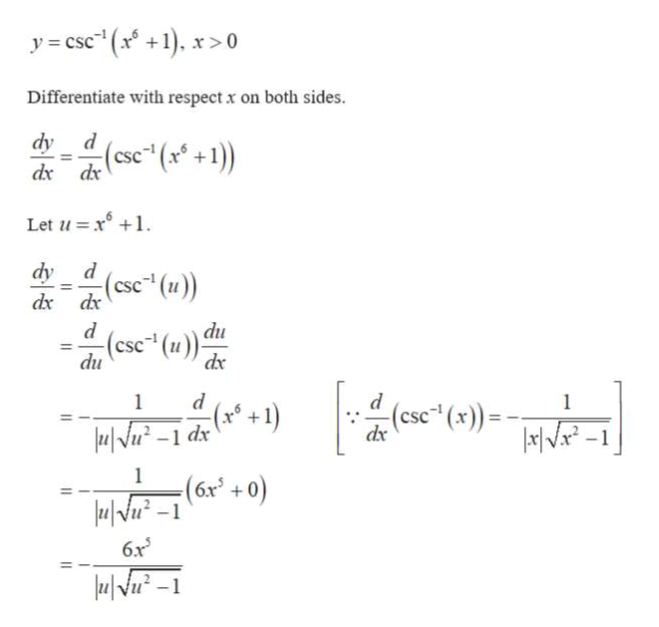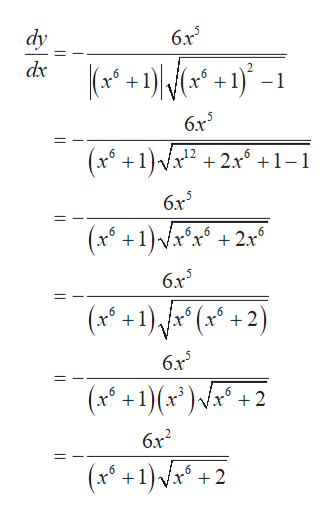# Find the derivative of y with respect to xy = csc^(-1)(x^6 + 1), x> 0

Question
2 views

Find the derivative of y with respect to x

y = csc^(-1)(x^6 + 1), x> 0

check_circle

Step 1

Find the derivative of the given function.help_outlineImage Transcriptionclosey= cse (x +1), x>0 Differentiate with respect x on both sides. dy d dx dx (se (x +1) Let u x dy dx dx d (sc (u)) (ese (u) du dx du d (I+) ap I-"N (6x* + 0) dx 1 (0+9N 6x3 fullscreen
Step 2

Substitute u in terms of x in order to...help_outlineImage Transcriptionclosedy 6x3 dx +1)-1 6x5 (x +1)D 6 2x11 6x5 (x +1)r*® 6 6 2x6 6x5 (x+1)\hx* (x* + 2) 6 6x5 (x+1)(x*)a* + 6 6 6x2 (x ) 2 fullscreen

### Want to see the full answer?

See Solution

#### Want to see this answer and more?

Solutions are written by subject experts who are available 24/7. Questions are typically answered within 1 hour.*

See Solution
*Response times may vary by subject and question.
Tagged in

### Derivative